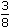# Aptitude - Partnership

### Exercise :: Partnership - Data Sufficiency 3

Each of these questions is followed by three statements. You have to study the question and all the three statements given to decide whether any information provided in the statement(s) is redundant and can be dispensed with while answering the given question.

1.

 Three friends, P, Q and R started a partnership business investing money in the ratio of 5 : 4 : 2 respectively for a period of 3 years. What is the amount received by P as his share profit? I. Total amount invested in the business in Rs. 22,000. II. Profit earned at the end of 3 years isof the total investment. III. The average amount of profit earned per year is Rs. 2750.

 A. I or II or III B. Either III only, or I and II together C. Any two of the three D. All I, II and III are required. E. None of these

Explanation:

 I and II give, profit after 3 years = Rs.3 x 22000= Rs. 8250. 8

From III also, profit after 3 years = Rs. (2750 x 3) = Rs. 8250.P's share = Rs.8250 x 5= Rs. 3750. 11

Thus, (either III is redundant [or] I and II are redundant).Correct answer is (B).